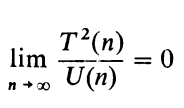# I. Introduction.

In his celebrated paper , A. M. Turing investigated the computability of sequences (functions) by mechanical procedures and showed that the setofsequences can be partitioned into computable and noncomputable sequences. One finds, however, that some computable sequences are very easy to compute whereas other computable sequences seem to have an inherent complexity that makes them difficult to compute. In this paper, we investigate a scheme of classifying sequences according to how hard they are to compute. This scheme puts a rich structure on the computable sequences and a variety of theorems are established. Furthermore, this scheme can be generalized to classify numbers, functions, or recognition problems according to their computational complexity.

The computational complexity of a sequence is to be measured by how fast a multitape Turing machine can print out the terms of the sequence. This particular abstract model of a computing device is chosen because much of the work in this area is stimulated by the rapidly growing importance of computation through the use of digital computers, and all digital computers in a slightly idealized form belong to the class of multitape Turing machines. More specifically, if Tin) is a computable, monotone increasing function of positive integers into positive integers and if a is a (binary) sequence, then we say that a is in complexity class ST or that a is T-computable if and only if there is a multitape Turing machine 3~ such that 3~ computes the nth term of a. within Tin) operations. Each set ST is recursively enumerable and so no class ST contains all computable sequences. On the other hand, every computable a is contained in some complexity class ST. Thus a hierarchy of complexity classes is assured. Furthermore, the classes are independent of time scale or of the speed of the components from which the machines could be built, as there is a “speed-up” theorem which states that ST = SkT for positive numbers k.

As corollaries to the speed-up theorem, there are several limit conditions which establish containment between two complexity classes. This is contrasted later with the theorem which gives a limit condition for noncontainment. One form of this result states that if (with minor restrictions)then S,; properly contains ST. The intersection of two classes is again a class. The general containment problem, however, is recursively unsolvable.

One section is devoted to an investigation as to how a change in the abstract machine model might affect the complexity classes. Some of these are related by a “square law,” including the one-tape-multitape relationship: that is if a is T-computable by a multitape Turing machine, then it is T2-computable by a single tape Turing machine. It is gratifying, however, that some of the more obvious variations do not change the classes.

The complexity of rational, algebraic, and transcendental numbers is studied in another section. There seems to be a good agreement with our intuitive notions, but there are several questions still to be settled.

There is a section in which generalizations to recognition problems and functions are discussed. This section also provides the first explicit “impossibility” proof, by describing a language whose “words” cannot be recognized in real-time [T(n) = n] .

The final section is devoted to open questions and problem areas. It is our conviction that numbers and functions have an intrinsic computational nature according to which they can be classified, as shown in this paper, and that there is a good opportunity here for further research.

For background information about Turing machines, computability and related topics, the reader should consult . “Real-time” computations (i.e., T(n) = n) were first defined and studied in . Other ways of classifying the complexity of a computation have been studied in  and , where the complexity is defined in terms of the amount of tape used.

Complete text here.